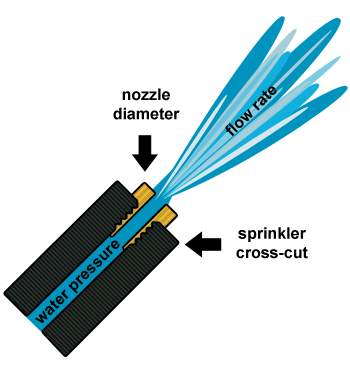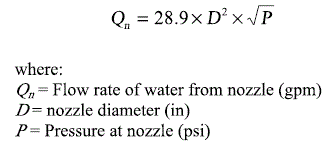# Nozzle Requirements

Find the nozzle requirements for a given pressure or flow rate.## Nozzle Discharge

This form is used to calculate the flow rate of a nozzle given the pressure.

Pressure:
Diameter:
Flow Rate:

## Nozzle Diameter Size

This form is used to calculate the needed diameter of a nozzle for a specific pressure and flow rate.

Pressure:
Flow Rate:
Diameter:

## The Equation

This calculator uses this formula to determine the Flow rate given a nozzle diameter and pressure. Or the required nozzle diameter to achieve a specified flow rate given the pressure.WSU Prosser - IAREC, 24106 N Bunn Rd, Prosser WA 99350-8694, 509-786-2226,